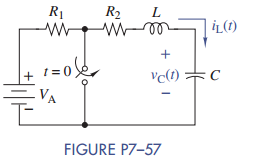Create an Account

Home / Questions / The switch in Figure P7–57 has been open for a long time and is closed at t = 0. The circuit...

The switch in Figure P7–57 has been open for a long time and is closed at t = 0. The circuit...

The switch in Figure P7–57 has been open for a long time and is closed at t = 0. The circuit parameters are L = 4 H, C = 1 μF, R1 = 2.2 kΩ, R2 = 3.3 kΩ, and VA = 12 V.

(a) Find vC (t) and iL(t) for t ≥ 0.

(b) Is the circuit overdamped, critically damped, or underdamped?

(c) Use Multisim to validate your results.Jul 28 2020 View more View LessSubscribe To Get Solution